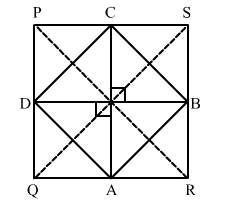# The quadrilateral formed by joining the midpoints of the sides of a quadrilateral ABCD,

Question:

The quadrilateral formed by joining the midpoints of the sides of a quadrilateral ABCD, taken in order, is a rhombus, if
(a) ABCD is a Parallelogram
(b) ABCD is rhombus
(c) diagonals of ABCD are equal
(4) diagonals of ABCD are perpendicular to each other.

Solution:

Given:The quadrilateral ABCD is a rhombus.

So, the sides ABBCCD and AD are equal.

Now, in $\triangle P Q S$, we have

$D C=\frac{1}{2} Q S$     (Using mid-point theorem)          ...(1)

Similarly, in $\triangle P S R$,

$B C=\frac{1}{2} P R$                  ..(2)

As, BC = DC

$\Rightarrow \frac{1}{2} Q S=\frac{1}{2} P R$    [From (1) and (2)]

So, QS = PR

Thus, the diagonals of PQRS are equal.

Hence, the correct option is (c).